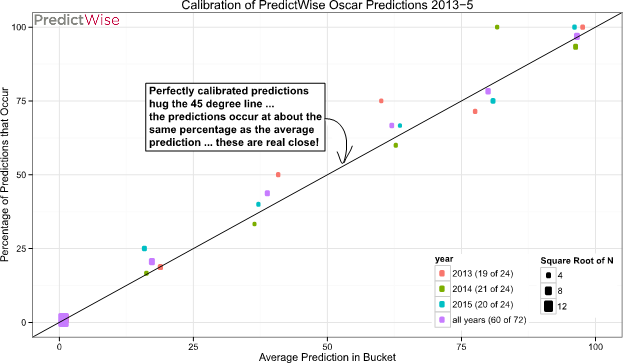Our Oscar predictions have been 19 for 24, 21 for 24, and 20 for 24 over the last three years, in the binary outcome space (i.e., the most likely candidate won the Oscar). Of the 12 “misses” 11 have been the second most likely and one has been the third most likely. But, our predictions are not probabilities for a reason; if we only cared about which candidate was the most likely and not how likely, we would not bother calibrating the difference!

What we are most proud of is the calibration of the Oscar predictions. In the 72 categories (24 per year) we have forecasted in the last three years, the average forecast for the leading candidate was 82%. Thus, on average, we expected to “win” a category 82% of the time and “lose” a category 18% of the time. Thus, 0.82*72 = 59 “wins” and 0.18*72 = 13 “losses” in expectation. Our 60 “wins” is pretty well calibrated!

A better way to think about calibration is to look at the 365 predictions we have made in the last three years. Of course, the predictions are not independent of each other (only one candidate can win in any category/year combination), but with five candidates in a category (except up to 9 in Picture and 3 in Makeup and Hairstyling) it is reasonable to use all predictions in testing calibration. On the x-axis we round each prediction into six buckets and on the y-axis we plot the percentage of predictions in that bucket that actually occur.In an ideally calibrated set of predictions, the answers would all lie on the 45 degree line; if the average prediction is 20% in a group of predictions, it should occur 20% of the time. All three years are extremely well calibrated.

The final Oscar predictions are prediction market-based; the model is in this paper.